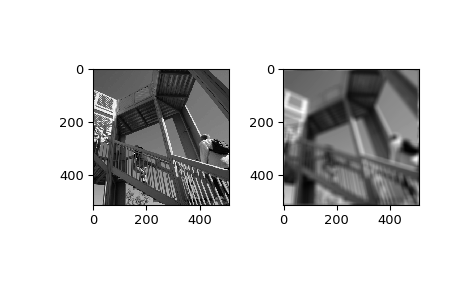# scipy.ndimage.fourier_uniform¶

scipy.ndimage.fourier_uniform(input, size, n=-1, axis=-1, output=None)[source]

Multi-dimensional uniform fourier filter.

The array is multiplied with the fourier transform of a box of given size.

Parameters: input : array_like The input array. size : float or sequence The size of the box used for filtering. If a float, size is the same for all axes. If a sequence, size has to contain one value for each axis. n : int, optional If n is negative (default), then the input is assumed to be the result of a complex fft. If n is larger than or equal to zero, the input is assumed to be the result of a real fft, and n gives the length of the array before transformation along the real transform direction. axis : int, optional The axis of the real transform. output : ndarray, optional If given, the result of filtering the input is placed in this array. None is returned in this case. fourier_uniform : ndarray or None The filtered input. If output is given as a parameter, None is returned.

Examples

>>> from scipy import ndimage, misc
>>> import numpy.fft
>>> import matplotlib.pyplot as plt
>>> fig, (ax1, ax2) = plt.subplots(1, 2)
>>> plt.gray()  # show the filtered result in grayscale
>>> ascent = misc.ascent()
>>> input_ = numpy.fft.fft2(ascent)
>>> result = ndimage.fourier_uniform(input_, size=20)
>>> result = numpy.fft.ifft2(result)
>>> ax1.imshow(ascent)
>>> ax2.imshow(result.real)  # the imaginary part is an artifact
>>> plt.show()